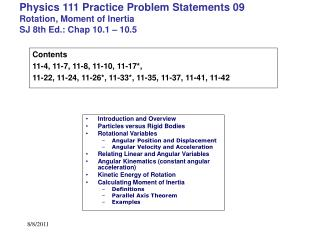# Physics 111 Practice Problem Statements 09 Rotation, Moment of Inertia SJ 8th Ed.: Chap 10.1 – 10.5 - PowerPoint PPT PresentationDownload PresentationPhysics 111 Practice Problem Statements 09 Rotation, Moment of Inertia SJ 8th Ed.: Chap 10.1 – 10.5

Physics 111 Practice Problem Statements 09 Rotation, Moment of Inertia SJ 8th Ed.: Chap 10.1 – 10.5Download Presentation## Physics 111 Practice Problem Statements 09 Rotation, Moment of Inertia SJ 8th Ed.: Chap 10.1 – 10.5

- - - - - - - - - - - - - - - - - - - - - - - - - - - E N D - - - - - - - - - - - - - - - - - - - - - - - - - - -
##### Presentation Transcript

1. Introduction and Overview Particles versus Rigid Bodies Rotational Variables Angular Position and Displacement Angular Velocity and Acceleration Relating Linear and Angular Variables Angular Kinematics (constant angular acceleration) Kinetic Energy of Rotation Calculating Moment of Inertia Definitions Parallel Axis Theorem Examples Physics 111 Practice Problem Statements 09Rotation, Moment of InertiaSJ 8th Ed.: Chap 10.1 – 10.5 Contents 11-4, 11-7, 11-8, 11-10, 11-17*, 11-22, 11-24, 11-26*, 11-33*, 11-35, 11-37, 11-41, 11-42

2. PROBLEM 11-4 The angular position of a point on the rim of a rotating wheel is given by q = 4.0t - 3.0t2 + t3, where q is in radians and t is in seconds. What are the angular velocities at (a) t = 2.0 s and (b) t = 4.0 s? (c) What is the average angular acceleration for the time interval that begins at t = 2.0 s and ends at t = 4.0 s? What are the instantaneous angular accelerations at (d) the beginning and (e) the end of this time interval?

3. PROBLEM 11-7 A diver makes 2.5 revolutions on the way from a 10-m-high platform to the water. Assuming zero initial vertical velocity, find the diver's average angular velocity during a dive.

4. PROBLEM 11-8 The angular speed of an automobile engine is increased at a constant rate from 1200 rev/min to 3000 rev/min in 12 s. (a) What is its angular acceleration in revolutions per minute-squared? (b) How many revolutions does the engine make during this 12 s interval?

5. PROBLEM 11-10: A disk, initially rotating at 120 rad/s, is slowed down with a constant angular acceleration of magnitude 4.0 rad/s/s. (a) How much time does the disk take to stop? (b) Through what angle does the disk rotate during that time?

6. PROBLEM 11-17*: A flywheel turns through 40 rev as it slows from an angular speed of 1.5 rad/s to a stop. (a) Assuming a constant angular acceleration, find the time for it to come to rest. (b) What is its angular acceleration? (c) How much time is required for it to complete the first 20 of the 40 revolutions?

7. PROBLEM 11-22*: A flywheel with a diameter of 1.20 m is rotating at an angular speed of 200 rev/min. (a) What is the angular speed of the flywheel in radians per second? (b) What is the linear speed of a point on the rim of the flywheel? (c) What constant angular acceleration (in revolutions per minute-squared) will increase the wheel's angular speed to 1000 rev/min in 60 s? (d) How many revolutions does the wheel make during that 60 s?

8. PROBLEM 11-24: What are the magnitudes of (a) the angular velocity, (b) the radial acceleration, and (c) the tangential acceleration of a spaceship taking a circular turn of radius 3220 km at a speed of 29000 km/h?

9. PROBLEM 11-26*: The flywheel of a steam engine runs with a constant angular velocity of 150 rev/min. When steam is shut off, the friction of the bearings and of the air stops the wheel in 2.2 h. (a) What is the constant angular acceleration, in revolutions per minute-squared, of the wheel during the slowdown? (b) How many rotations does the wheel make before stopping? (c) At the instant the flywheel is turning at 75 rev/min, what is the tangential component of the linear acceleration of a flywheel particle that is 50 cm from the axis of rotation? (d) What is the magnitude of the net linear acceleration of the particle in (c)?

10. PROBLEM 11-33*: Calculate the rotational inertia of a wheel that has a kinetic energy of 24400 J when rotating at 602 rev/min.

11. PROBLEM 11-35: Two uniform solid cylinders, each rotating about its central (longitudinal) axis, have the same mass of 1.25 kg and rotate with the same angular speed of 235 rad/s, but they differ in radius. What is the rotational kinetic energy of (a) the smaller cylinder, of radius 0.25 m, and (b) the larger cylinder, of radius 0.75 m?

12. PROBLEM 11-37: In Figure 11-34, two particles, each with mass m, are fastened to each other, and to a roation axis at O, by two thin rods, each with length d and mass M. The combination rotates around the rotation axis with angular velocity w. In terms of these symbols, and measured about O, what are the combination's (a) rotational inertia and (b) kinetic energy?

13. PROBLEM 11-41: The uniform solid block in Fig. 11-36has mass M and edge lengths a, b, and c. Calculate its rotational inertia about an axis through one corner and perpendicular to the large faces.

14. PROBLEM 11-42: The masses and coordinates of four particles are as follows: 50 g, x = 2.0 cm, y = 2.0 cm; 25 g, x = 0, y = 4.0 cm; 25 g, x = -3.0 cm, y = -3.0 cm; 30 g, x = -2.0 cm, y = 4.0 cm. What are the rotational inertias of this collection about the (a) x, (b) y, and (c) z axes? (d) Suppose the answers to (a) and (b) are A and B, respectively. Then what is the answer to (c) in terms of A and B?